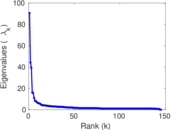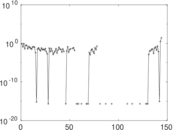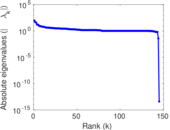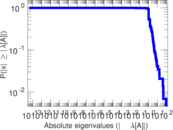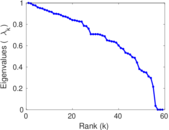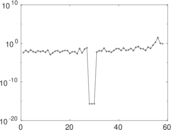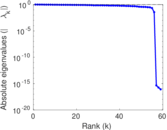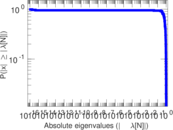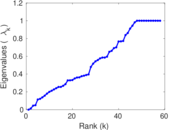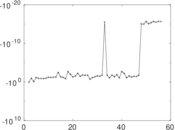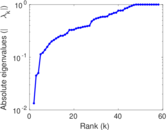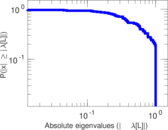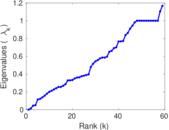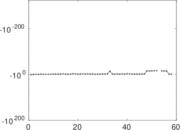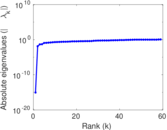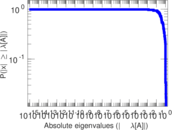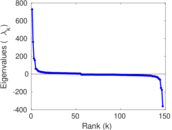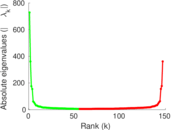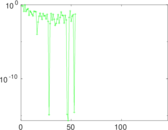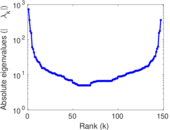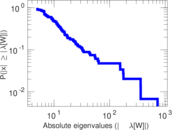Wikibooks edits (fy)

This is the bipartite edit network of the Western Frisian Wikibooks. It contains users and pages from the Western Frisian Wikibooks, connected by edit events. Each edge represents an edit. The dataset includes the timestamp of each edit.

 Code bfy Internal name edit-fywikibooks Name Wikibooks edits (fy) Data source http://dumps.wikimedia.org/ AvailabilityDataset is available for download Consistency checkDataset passed all tests Category Authorship network Dataset timestamp 2017-10-20 Node meaning User, article Edge meaning Edit Network formatBipartite, undirected Edge typeUnweighted, multiple edges Temporal dataEdges are annotated with timestamps

Statistics

 Size n = 739 Left size n1 = 147 Right size n2 = 592 Volume m = 1,173 Unique edge count m̿ = 722 Wedge count s = 24,660 Claw count z = 1,213,605 Cross count x = 50,270,189 Square count q = 405 4-Tour count T4 = 103,808 Maximum degree dmax = 204 Maximum left degree d1max = 204 Maximum right degree d2max = 138 Average degree d = 3.174 56 Average left degree d1 = 7.979 59 Average right degree d2 = 1.981 42 Fill p = 0.008 296 56 Average edge multiplicity m̃ = 1.624 65 Size of LCC N = 486 Diameter δ = 12 50-Percentile effective diameter δ0.5 = 3.622 76 90-Percentile effective diameter δ0.9 = 5.891 68 Median distance δM = 4 Mean distance δm = 4.280 92 Gini coefficient G = 0.663 918 Balanced inequality ratio P = 0.235 720 Left balanced inequality ratio P1 = 0.184 996 Right balanced inequality ratio P2 = 0.329 923 Relative edge distribution entropy Her = 0.848 108 Power law exponent γ = 5.059 68 Tail power law exponent γt = 2.631 00 Tail power law exponent with p γ3 = 2.631 00 p-value p = 0.008 000 00 Left tail power law exponent with p γ3,1 = 2.111 00 Left p-value p1 = 0.280 000 Right tail power law exponent with p γ3,2 = 4.131 00 Right p-value p2 = 0.782 000 Degree assortativity ρ = −0.164 504 Degree assortativity p-value pρ = 8.876 26 × 10−6 Spectral norm α = 90.508 0 Algebraic connectivity a = 0.013 337 9 Spectral separation |λ1[A] / λ2[A]| = 2.048 03 Controllability C = 441 Relative controllability Cr = 0.604 938

Plots

Fruchterman–Reingold graph drawing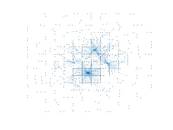Degree distribution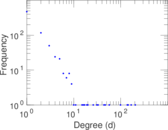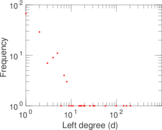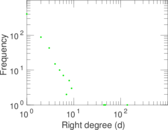Cumulative degree distribution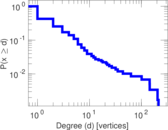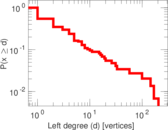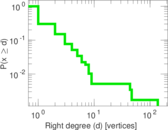Lorenz curve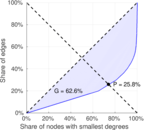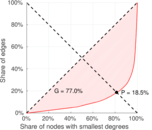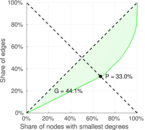Spectral distribution of the adjacency matrix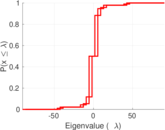Spectral distribution of the normalized adjacency matrix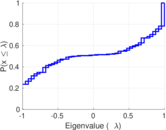Spectral distribution of the Laplacian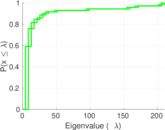Spectral graph drawing based on the adjacency matrixSpectral graph drawing based on the Laplacian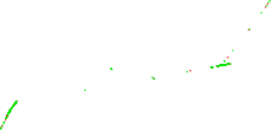Spectral graph drawing based on the normalized adjacency matrix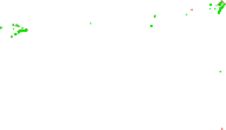Degree assortativity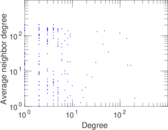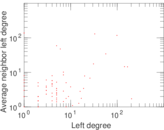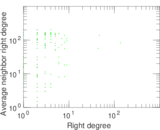Zipf plot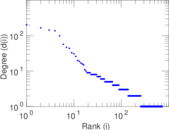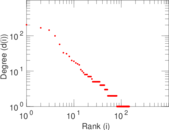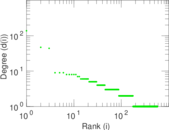Hop distribution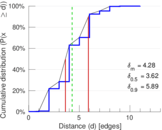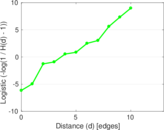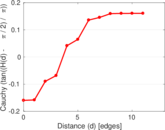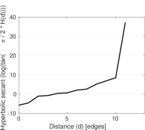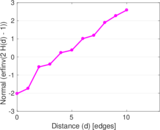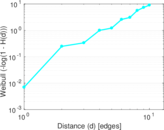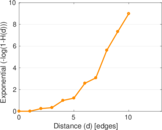Double Laplacian graph drawing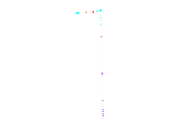Delaunay graph drawing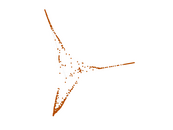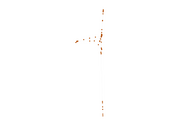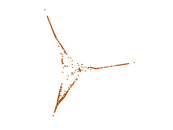Edge weight/multiplicity distribution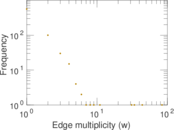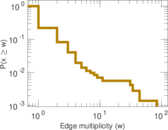Temporal distribution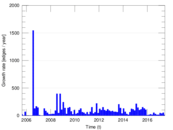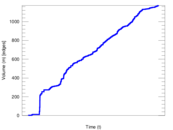Temporal hop distribution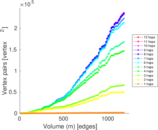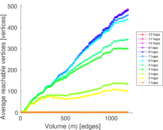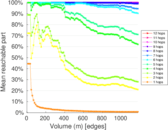Diameter/density evolution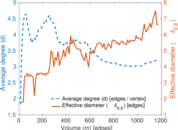Matrix decompositions plots